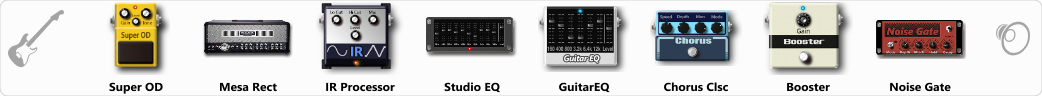# Dimebag Style

Discussion in 'ToneLib-GFX presets' started by Russ, Sep 24, 2019.

1. Dimebag Style

GFX version: 3.0

Preset name: Dimebag Style
Optimized for: Phones/Speaker

Effects chain:Sounds great for me, hopefully you guys enjoy it to.

Effect: "Super OD" (Overdrive / Distortion), active - "yes"
"Drive" = 28
"Tone" = 95
"Level" = 95

Effect: "Mesa Rect" (Amp simulators), active - "yes"
"Gain" = 51
"Bass" = 17
"Middle" = 30
"Treble" = 77
"Presence" = 50
"Master" = 50
"Output" = 50
"Level (dB)" = 5

Effect: "IR Processor" (Dynamics / Filter), active - "yes"
"IR" = PanteraVulgarDisplayOfPower
"Low Cut (Hz)" = 17
"Hi Cut (kHz)" = 13.0
"Mix" = 100
"Level (dB)" = 0

Effect: "Studio EQ" (Dynamics / Filter), active - "yes"
"31 Hz" = -1
"62 Hz" = -2
"125 Hz" = 1
"250 Hz" = -1
"500 Hz" = -7
"1 kHz" = -1
"2 kHz" = -2
"4 kHz" = 1
"8 kHz" = 6
"16 kHz" = 0
"above 16 kHz" = 0
"Level (dB)" = 0

Effect: "GuitarEQ" (Dynamics / Filter), active - "yes"
"160 Hz" = 0
"400 Hz" = 4
"800 Hz" = 4
"1.6 kHz" = 4
"3.2 kHz" = -1
"6.4 kHz" = 5
"12 kHz" = -2
"Level (dB)" = 0

Effect: "Chorus Clsc" (Modulation / Sfx), active - "yes"
"Speed" = 4.9
"Depth" = 49
"Center" = 1.0
"Mode" = Stereo

Effect: "Booster" (Dynamics / Filter), active - "yes"
"Gain" = 58

Effect: "Noise Gate" (Dynamics / Filter), active - "yes"
"Detect" = Gtr In
"Mode" = Manual
"Depth" = 100
"Threshold" = 64
"Attack" = 0
"Hold" = 5
"Decay" = 250

Note: This is a ToneLib-GFX preset file, you will need to download and install the ToneLib-GFX software to use the preset.

File size:
3.5 KB
Views:
8,157